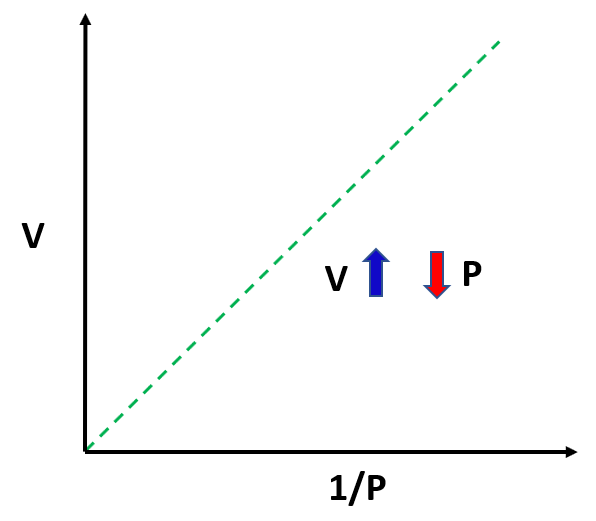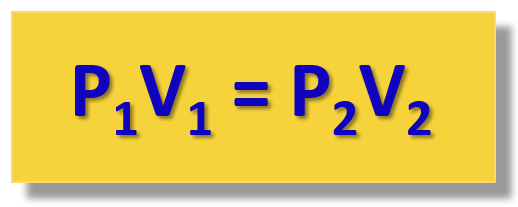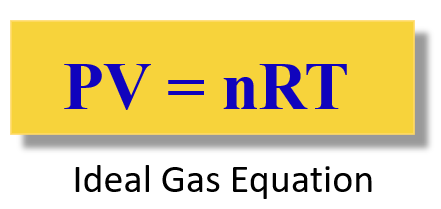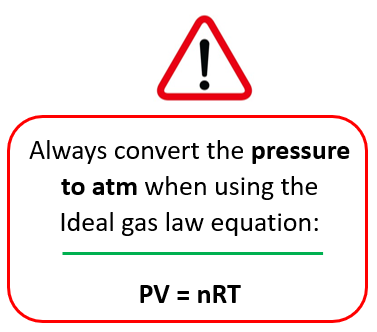## General Chemistry

In this post, we will discuss one of the gas laws using an illustration with a pump filled with some gas and a freely moving plunger and by changing the gas parameters determine their correlation.

# Boyle’s Law

Boyle’s Law shows the correlation between the pressure and the volume of a gas. To see how these two parameters change based on one another, suppose we need to alter them. Let’s start with the volume. If we push down the plunger, it’s evident that the volume of the gas is decreasing.  Now, as push down, the volume is decreasing and because the gas molecules have less space, the pressure is increasing:The observation is that the volume of a fixed quantity of gas at constant temperature is inversely proportional to its pressure.This relationship can be written as:

${\rm{V}} \sim \,\frac{{\rm{1}}}{{\rm{P}}}$

To bring in the equal sign, we introduce a constant:

PV = constant

This can be explained using the example of a car dealership income. The income depends on the number of sales which we can represent as:

Income  number of cars

However, we cannot say income = number of cars sold, so to switch an equal sign, we need to introduce a constant. This can be the price of the car transforming the equation to:

Income  = price x number of cars

So, for the gas pressure and volume, we are not interested too much in the constant, but rather in its linkage of pressure and volume at positions 1 and 2. Because the P x V product is constant, we can write that:

P1V1 = constant = P2V2

orThis is the practical implication of the Boyle’s law that is used for solving gas problems.

For example,

The pressure of a gas is 2.30 atm in a 1.80 L container. Calculate the final pressure of the gas if the volume is decreased to 1.20 liters.

First, write down what you have and what needs to be determined. If nothing is mentioned about any parameter, for example, the moles and the temperature in this case, it is assumed that they are constant, so you don’t need to worry about them.

P1 = 2.30 atm

V1 = 1.80 L

V2 = 1.20 L

P2 = ?

${{\rm{P}}_{\rm{1}}}{{\rm{V}}_{\rm{1}}}\; = \;{{\rm{P}}_{\rm{2}}}{{\rm{V}}_{\rm{2}}}$

Now, rearrange to calculate P2:

${{\rm{P}}_{\rm{2}}}\; = \;\frac{{{{\rm{P}}_{\rm{1}}}{{\rm{V}}_{\rm{1}}}}}{{{{\rm{V}}_{\rm{2}}}}}$

${{\rm{P}}_{\rm{2}}}\; = \;\frac{{{\rm{2}}{\rm{.30}}\;{\rm{atm}}\;{\rm{ \times 1}}{\rm{.80}}\;\cancel{{\rm{L}}}}}{{{\rm{1}}{\rm{.20}}\;\cancel{{\rm{L}}}}}\;{\rm{ = }}\;{\rm{3}}{\rm{.45}}\;{\rm{atm}}$

# The Ideal Gas Law

There are other gas laws such as the Charles’ law, Avogadro’s law etc., and they are all together summarized in the ideal gas law equation. Unlike the individual gas laws, the ideal gas law links all the variables into one equation:The R is called the ideal gas constant. Although it has different values and units, you will mostly be using this:

$R\;{\rm{ = }}\;{\rm{0}}{\rm{.08206}}\;\frac{{{\rm{L}} \cdot {\rm{atm}}}}{{{\rm{mol}} \cdot {\rm{K}}}}$

Remember, to change the pressure to atm when the ideal gas law equation is used! This is because the units of R contain atm when the 0.08206 value is used. And this is what most problems in this chapter use.# How do I know which gas law to use?

Once you cover all the gas laws, it sometimes becomes challenging to choose the appropriate one when solving problems. For this, there is what is called the combined gas law and as long as you remember it, you do not need to remember all the gas laws to solve a problem.

Let’s keep it for another article because there is quite a lot of information in this one.

Check Also

### 2 thoughts on “<strong>Boyle’s Law</strong>”

1.Im pretty sure I’m going to subscribe to your 1 month deal. But I was wondering if you recommend any other books that might help me get through this general chemistry?
Or do you have a book that I could buy?

•